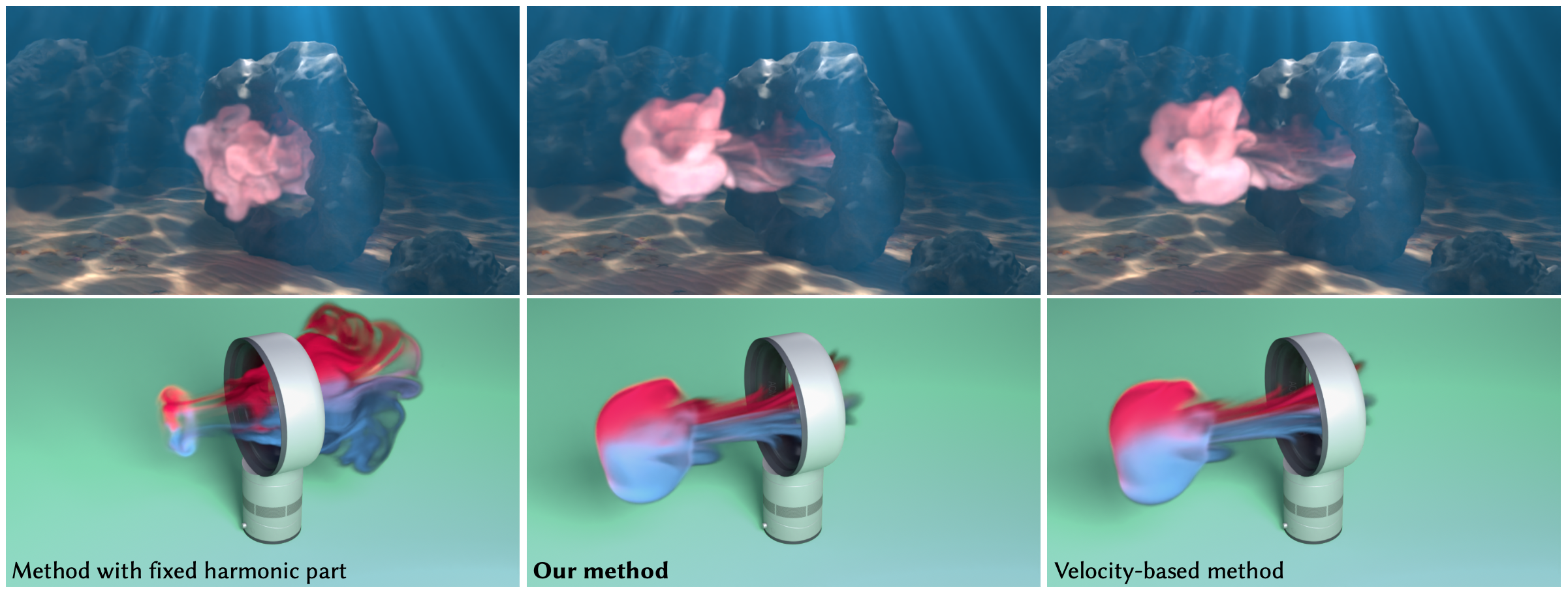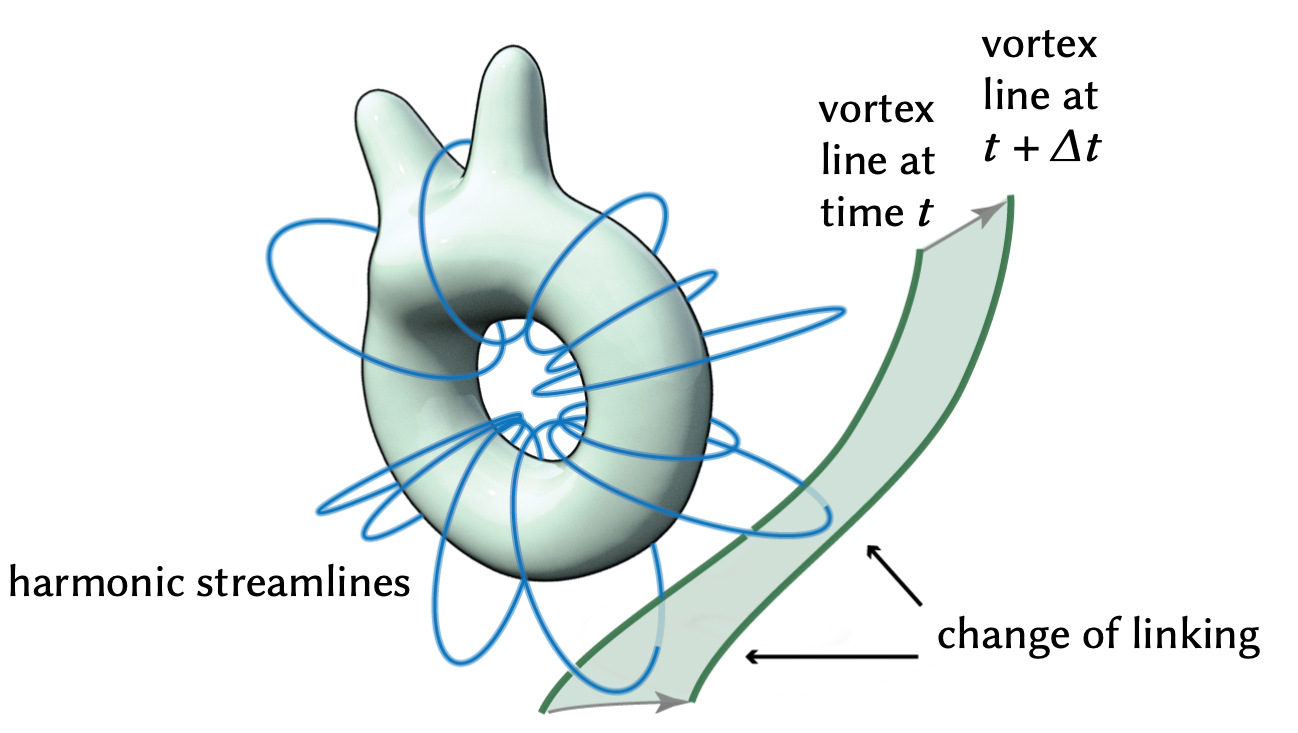# Fluid Cohomology

ACM Transactions on Graphics (SIGGRAPH 2023)## Abstract

The vorticity-streamfunction formulation for incompressible inviscid fluids is the basis for many fluid simulation methods in computer graphics, including vortex methods, streamfunction solvers, spectral methods, and Monte Carlo methods. We point out that current setups in the vorticity-streamfunction formulation are insufficient at simulating fluids on general non-simply-connected domains. This issue is critical in practice, as obstacles, periodic boundaries, and nonzero genus can all make the fluid domain multiply connected. These scenarios introduce nontrivial cohomology components to the flow in the form of harmonic fields. The dynamics of these harmonic fields have been previously overlooked. In this paper, we derive the missing equations of motion for the fluid cohomology components. We elucidate the physical laws associated with the new equations, and show their importance in reproducing physically correct behaviors of fluid flows on domains with general topology.